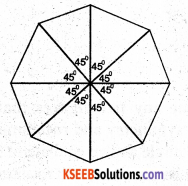# KSEEB Solutions for Class 7 Maths Chapter 14 Symmetry Ex 14.3

Students can Download Chapter 14 Symmetry Ex 14.3, Question and Answers, Notes Pdf, KSEEB Solutions for Class 7 Maths, Karnataka State Board Solutions help you to revise complete Syllabus and score more marks in your examinations.

## Karnataka State Syllabus Class 7 Maths Chapter 14 Symmetry Ex 14.3

Question 1.
Name any two figures that have both line symmetry and rotational symmetry.
Solution:
The circle and an equilateral triangle have both line symmetry and rotational symmetry.

Question 2.
Draw, wherever possible, a rough sketch of

i) a triangle with both line and rotational symmetries of order more than 1.
Solution:
An equilateral triangle has 3 lines of symmetry and rotational symmetry of order 3.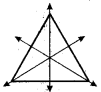ii) a triangle with only symmetry and to rotational symmetry of order more than 1.
Solution: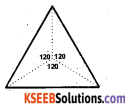Isosceles triangle has only one line symmetry but no rotational symmetry of order more than 1.

iii) a quadrilateral with a rotational symmetry of order more than 1 but not a line symmetry.
Solution:
A parallelogram has no line symmetry but has a rotational symmetry of order 2.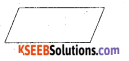iv) a quadrilateral with line symmetry but not a rotational symmetry of order more than 1.
Solution:
An isosceles trapezium has one line of symmetry of order more than 1.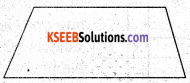Question 3.
If a figure has two or more lines of symmetry, should it have rotational symmetry of order more than 1 ?
Solution:
Yes

Question 4.
Fill in the blanks :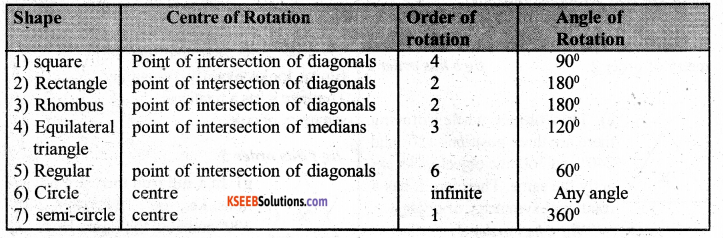Question 5.
Name the quadrilaterals which have both line and rotational symmetry of order more than 1.
Solution:
i) Square
ii) RectangleQuestion 6.
After rotating by 600 about a centre, a figure looks exactly the same as its original position. At what other angles will this happen for the figure ?
Solution:
The figure is regular hexagon. The other angles are 120°, 180°, 240°, 300°and 360°.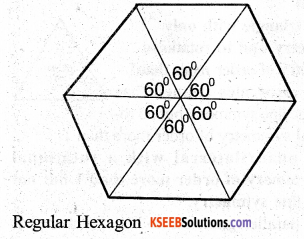Question 7.
Can we have a rotational symmetry of order more than 1 whose angle of rotation is
Solution:
i) 45° ?
yes

ii) 17° ?
No, It is possible